# The normal healing of wounds can be modeled by an exponential function. If A0 represents the original area of the wound, and if A equals the area of the wound, the the function A(n)=A0e-0.35n describes the area of a wound after n days following an injury when no infection is present to retard the healing. Suppose that a wound initially had an area of 100 sqaure millimeters.a) If healing is taking place, after how many days will the wound be one-half of its original size?b) How long before the wound is 10% of its original size?

Question
37 views

The normal healing of wounds can be modeled by an exponential function. If A0 represents the original area of the wound, and if A equals the area of the wound, the the function A(n)=A0e-0.35n describes the area of a wound after n days following an injury when no infection is present to retard the healing. Suppose that a wound initially had an area of 100 sqaure millimeters.

a) If healing is taking place, after how many days will the wound be one-half of its original size?

b) How long before the wound is 10% of its original size?

check_circle

Step 1

Given that the function is: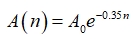where A0 is the original area of the wound and A(n) is the area of the wound after n days.

Step 2

(a) When area is one half of its original size, take: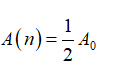So,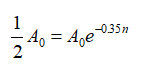A0, will cancel out from both the sides, we get: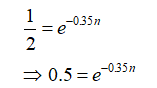Taking natural logarithm on both sides, we get: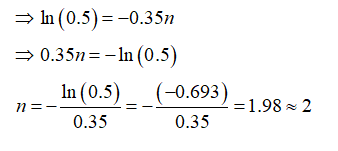Hence, the wound becomes one-half of its original size approximately after 2 days.

...

### Want to see the full answer?

See Solution

#### Want to see this answer and more?

Solutions are written by subject experts who are available 24/7. Questions are typically answered within 1 hour.*

See Solution
*Response times may vary by subject and question.
Tagged in